# Calculas

17 May 2017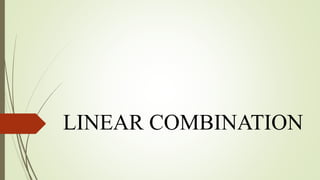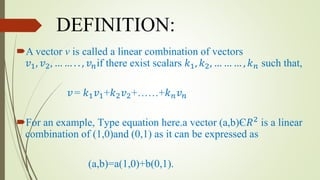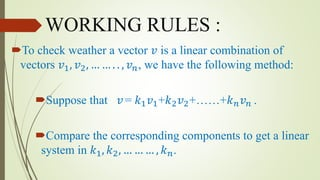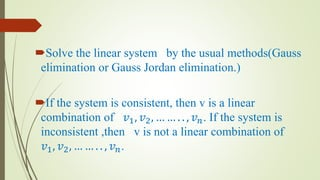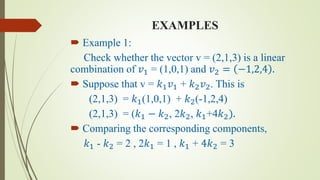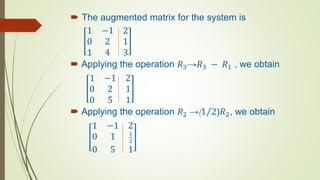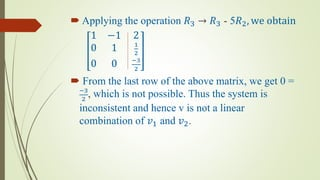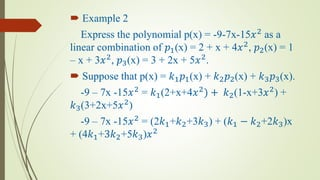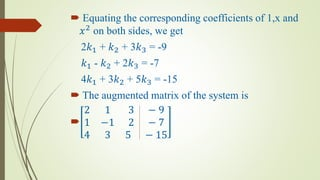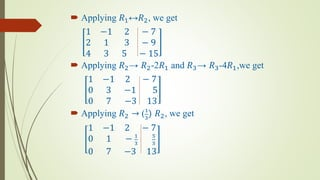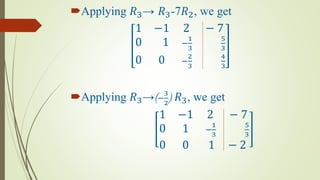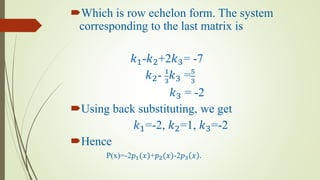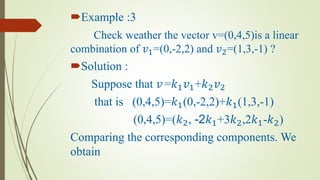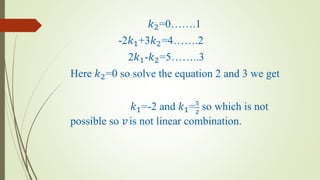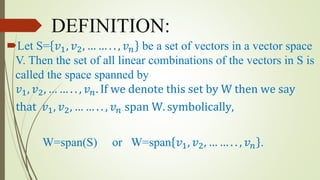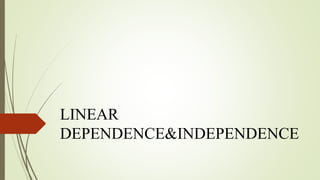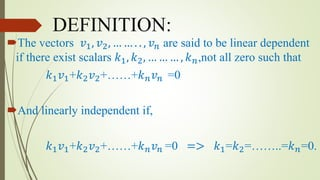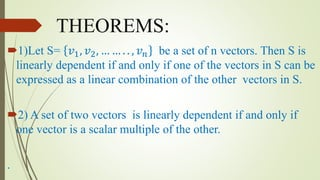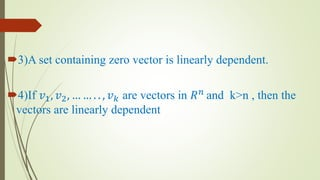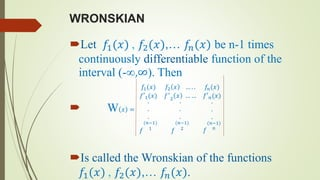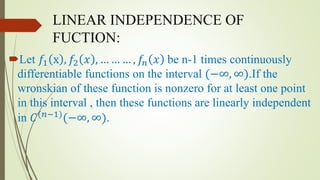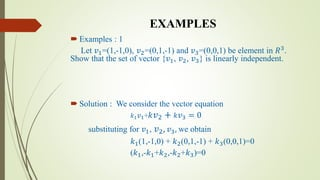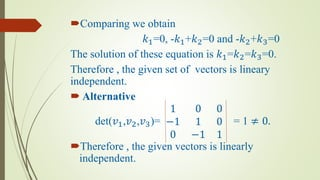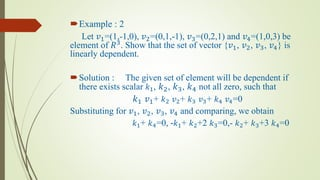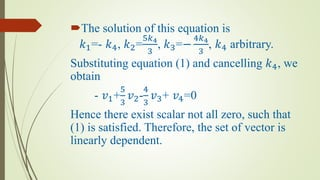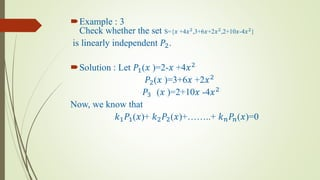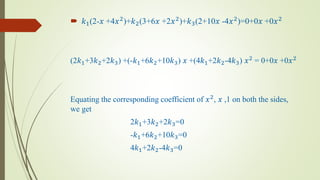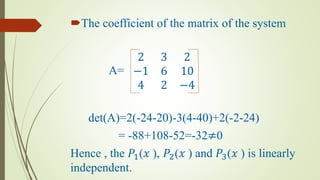1 sur 30

### Calculas

• 3. DEFINITION: A vector v is called a linear combination of vectors 𝑣1, 𝑣2, … … . . , 𝑣 𝑛if there exist scalars 𝑘1, 𝑘2, … … … , 𝑘 𝑛 such that, 𝑣= 𝑘1 𝑣1+𝑘2 𝑣2+……+𝑘 𝑛 𝑣 𝑛 For an example, Type equation here.a vector (a,b)Є𝑅2 is a linear combination of (1,0)and (0,1) as it can be expressed as (a,b)=a(1,0)+b(0,1).
• 4. WORKING RULES : To check weather a vector 𝑣 is a linear combination of vectors 𝑣1, 𝑣2, … … . . , 𝑣 𝑛, we have the following method: Suppose that 𝑣= 𝑘1 𝑣1+𝑘2 𝑣2+……+𝑘 𝑛 𝑣 𝑛 . Compare the corresponding components to get a linear system in 𝑘1, 𝑘2, … … … , 𝑘 𝑛.
• 5. Solve the linear system by the usual methods(Gauss elimination or Gauss Jordan elimination.) If the system is consistent, then v is a linear combination of 𝑣1, 𝑣2, … … . . , 𝑣 𝑛. If the system is inconsistent ,then v is not a linear combination of 𝑣1, 𝑣2, … … . . , 𝑣 𝑛.
• 6. EXAMPLES  Example 1: Check whether the vector v = (2,1,3) is a linear combination of 𝑣1 = (1,0,1) and 𝑣2 = −1,2,4 .  Suppose that v = 𝑘1 𝑣1 + 𝑘2 𝑣2. This is (2,1,3) = 𝑘1(1,0,1) + 𝑘2(-1,2,4) (2,1,3) = (𝑘1 − 𝑘2, 2𝑘2, 𝑘1+4𝑘2).  Comparing the corresponding components, 𝑘1 - 𝑘2 = 2 , 2𝑘1 = 1 , 𝑘1 + 4𝑘2 = 3
• 7.  The augmented matrix for the system is 1 −1 2 0 2 1 1 4 3  Applying the operation 𝑅3→𝑅3 − 𝑅1 , we obtain 1 −1 2 0 2 1 0 5 1  Applying the operation 𝑅2 →(1 2)𝑅2, we obtain 1 −1 2 0 1 1 2 0 5 1
• 8.  Applying the operation 𝑅3 → 𝑅3 - 5𝑅2, we obtain 1 −1 2 0 1 1 2 0 0 −3 2  From the last row of the above matrix, we get 0 = −3 2 , which is not possible. Thus the system is inconsistent and hence v is not a linear combination of 𝑣1 and 𝑣2.
• 9.  Example 2 Express the polynomial p(x) = -9-7x-15𝑥2 as a linear combination of 𝑝1(x) = 2 + x + 4𝑥2 , 𝑝2(x) = 1 – x + 3𝑥2 , 𝑝3(x) = 3 + 2x + 5𝑥2 .  Suppose that p(x) = 𝑘1 𝑝1(x) + 𝑘2 𝑝2(x) + 𝑘3 𝑝3(x). -9 – 7x -15𝑥2 = 𝑘1(2+x+4𝑥2 ) + 𝑘2(1-x+3𝑥2 ) + 𝑘3(3+2x+5𝑥2 ) -9 – 7x -15𝑥2 = (2𝑘1+𝑘2+3𝑘3) + (𝑘1 − 𝑘2+2𝑘3)x + (4𝑘1+3𝑘2+5𝑘3)𝑥2
• 10.  Equating the corresponding coefficients of 1,x and 𝑥2 on both sides, we get 2𝑘1 + 𝑘2 + 3𝑘3 = -9 𝑘1 - 𝑘2 + 2𝑘3 = -7 4𝑘1 + 3𝑘2 + 5𝑘3 = -15  The augmented matrix of the system is  2 1 3 − 9 1 −1 2 − 7 4 3 5 − 15
• 11.  Applying 𝑅1↔𝑅2, we get 1 −1 2 − 7 2 1 3 − 9 4 3 5 − 15  Applying 𝑅2→ 𝑅2-2𝑅1 and 𝑅3→ 𝑅3-4𝑅1,we get 1 −1 2 − 7 0 3 −1 5 0 7 −3 13  Applying 𝑅2 → (1 3 ) 𝑅2, we get 1 −1 2 − 7 0 1 − 1 3 5 3 0 7 −3 13
• 12. Applying 𝑅3→ 𝑅3-7𝑅2, we get 1 −1 2 − 7 0 1 − 1 3 5 3 0 0 − 2 3 4 3 Applying 𝑅3→(− 3 2 ) 𝑅3, we get 1 −1 2 − 7 0 1 − 1 3 5 3 0 0 1 − 2
• 13. Which is row echelon form. The system corresponding to the last matrix is 𝑘1-𝑘2+2𝑘3= -7 𝑘2- 1 3 𝑘3 =5 3 𝑘3 = -2 Using back substituting, we get 𝑘1=-2, 𝑘2=1, 𝑘3=-2 Hence P(x)=-2𝑝1(𝑥)+𝑝2(𝑥)-2𝑝3 𝑥 .
• 14. Example :3 Check weather the vector v=(0,4,5)is a linear combination of 𝑣1=(0,-2,2) and 𝑣2=(1,3,-1) ? Solution : Suppose that 𝑣=𝑘1 𝑣1+𝑘2 𝑣2 that is (0,4,5)=𝑘1(0,-2,2)+𝑘1(1,3,-1) (0,4,5)=(𝑘2, -2𝑘1+3𝑘2,2𝑘1-𝑘2) Comparing the corresponding components. We obtain
• 15. 𝑘2=0…….1 -2𝑘1+3𝑘2=4…….2 2𝑘1-𝑘2=5……..3 Here 𝑘2=0 so solve the equation 2 and 3 we get 𝑘1=-2 and 𝑘1=5 2 so which is not possible so 𝑣is not linear combination.
• 16. SPAN
• 17. DEFINITION: Let S= 𝑣1, 𝑣2, … … . . , 𝑣 𝑛 be a set of vectors in a vector space V. Then the set of all linear combinations of the vectors in S is called the space spanned by 𝑣1, 𝑣2, … … . . , 𝑣 𝑛. If we denote this set by W then we say that 𝑣1, 𝑣2, … … . . , 𝑣 𝑛 span W. symbolically, W=span(S) or W=span 𝑣1, 𝑣2, … … . . , 𝑣 𝑛 .
• 19. DEFINITION: The vectors 𝑣1, 𝑣2, … … . . , 𝑣 𝑛 are said to be linear dependent if there exist scalars 𝑘1, 𝑘2, … … … , 𝑘 𝑛,not all zero such that 𝑘1 𝑣1+𝑘2 𝑣2+……+𝑘 𝑛 𝑣 𝑛 =0 And linearly independent if, 𝑘1 𝑣1+𝑘2 𝑣2+……+𝑘 𝑛 𝑣 𝑛 =0 => 𝑘1=𝑘2=……..=𝑘 𝑛=0.
• 20. THEOREMS: 1)Let S= 𝑣1, 𝑣2, … … . . , 𝑣 𝑛 be a set of n vectors. Then S is linearly dependent if and only if one of the vectors in S can be expressed as a linear combination of the other vectors in S. 2) A set of two vectors is linearly dependent if and only if one vector is a scalar multiple of the other. .
• 21. 3)A set containing zero vector is linearly dependent. 4)If 𝑣1, 𝑣2, … … . . , 𝑣 𝑘 are vectors in 𝑅 𝑛 and k>n , then the vectors are linearly dependent
• 22. WRONSKIAN Let 𝑓1(𝑥) , 𝑓2(𝑥),… 𝑓𝑛(𝑥) be n-1 times continuously differentiable function of the interval (-∞,∞). Then  W 𝑥 = 𝑓1(𝑥) 𝑓2 𝑥 … . . 𝑓𝑛(𝑥) 𝑓′1(𝑥) 𝑓′ 2 𝑥 … … 𝑓′ 𝑛(𝑥) . . . 𝑓 (𝑛−1) 1 . . . 𝑓 (𝑛−1) 2 . . . 𝑓 (𝑛−1) 𝑛 Is called the Wronskian of the functions 𝑓1(𝑥) , 𝑓2(𝑥),… 𝑓𝑛(𝑥).
• 23. LINEAR INDEPENDENCE OF FUCTION: Let 𝑓1 x , 𝑓2 𝑥 , … … … , 𝑓𝑛 𝑥 be n-1 times continuously differentiable functions on the interval (−∞, ∞).If the wronskian of these function is nonzero for at least one point in this interval , then these functions are linearly independent in 𝐶(𝑛−1) (−∞, ∞).
• 24. EXAMPLES  Examples : 1 Let 𝑣1=(1,-1,0), 𝑣2=(0,1,-1) and 𝑣3=(0,0,1) be element in 𝑅3 . Show that the set of vector {𝑣1, 𝑣2, 𝑣3} is linearly independent.  Solution : We consider the vector equation 𝑘1 𝑣1+ 𝑘 𝑣2 + 𝑘 𝑣3 = 0 substituting for 𝑣1, 𝑣2, 𝑣3, we obtain 𝑘1(1,-1,0) + 𝑘2(0,1,-1) + 𝑘3(0,0,1)=0 (𝑘1,-𝑘1+𝑘2,-𝑘2+𝑘3)=0
• 25. Comparing we obtain 𝑘1=0, -𝑘1+𝑘2=0 and -𝑘2+𝑘3=0 The solution of these equation is 𝑘1=𝑘2=𝑘3=0. Therefore , the given set of vectors is lineary independent.  Alternative det(𝑣1,𝑣2,𝑣3)= 1 0 0 −1 1 0 0 −1 1 = 1 ≠ 0. Therefore , the given vectors is linearly independent.
• 26. Example : 2 Let 𝑣1=(1,-1,0), 𝑣2=(0,1,-1), 𝑣3=(0,2,1) and 𝑣4=(1,0,3) be element of 𝑅3. Show that the set of vector {𝑣1, 𝑣2, 𝑣3, 𝑣4} is linearly dependent. Solution : The given set of element will be dependent if there exists scalar 𝑘1, 𝑘2, 𝑘3, 𝑘4 not all zero, such that 𝑘1 𝑣1+ 𝑘2 𝑣2+ 𝑘3 𝑣3+ 𝑘4 𝑣4=0 Substituting for 𝑣1, 𝑣2, 𝑣3, 𝑣4 and comparing, we obtain 𝑘1+ 𝑘4=0, -𝑘1+ 𝑘2+2 𝑘3=0,- 𝑘2+ 𝑘3+3 𝑘4=0
• 27. The solution of this equation is 𝑘1=- 𝑘4, 𝑘2= 5𝑘4 3 , 𝑘3=− 4𝑘4 3 , 𝑘4 arbitrary. Substituting equation (1) and cancelling 𝑘4, we obtain - 𝑣1+ 5 3 𝑣2- 4 3 𝑣3+ 𝑣4=0 Hence there exist scalar not all zero, such that (1) is satisfied. Therefore, the set of vector is linearly dependent.
• 28. Example : 3 Check whether the set S={𝑥 +4𝑥2 ,3+6𝑥+2𝑥2 ,2+10𝑥-4𝑥2 } is linearly independent 𝑃2. Solution : Let 𝑃1(𝑥 )=2-𝑥 +4𝑥2 𝑃2(𝑥 )=3+6𝑥 +2𝑥2 𝑃3 (𝑥 )=2+10𝑥 -4𝑥2 Now, we know that 𝑘1 𝑃1(𝑥)+ 𝑘2 𝑃2(𝑥)+……..+ 𝑘 𝑛 𝑃𝑛(𝑥)=0
• 29.  𝑘1(2-𝑥 +4𝑥2 )+𝑘2(3+6𝑥 +2𝑥2 )+𝑘3(2+10𝑥 -4𝑥2 )=0+0𝑥 +0𝑥2 (2𝑘1+3𝑘2+2𝑘3) +(-𝑘1+6𝑘2+10𝑘3) 𝑥 +(4𝑘1+2𝑘2-4𝑘3) 𝑥2 = 0+0𝑥 +0𝑥2 Equating the corresponding coefficient of 𝑥2, 𝑥 ,1 on both the sides, we get 2𝑘1+3𝑘2+2𝑘3=0 -𝑘1+6𝑘2+10𝑘3=0 4𝑘1+2𝑘2-4𝑘3=0
• 30. The coefficient of the matrix of the system A= 2 3 2 −1 6 10 4 2 −4 det(A)=2(-24-20)-3(4-40)+2(-2-24) = -88+108-52=-32≠0 Hence , the 𝑃1(𝑥 ), 𝑃2(𝑥 ) and 𝑃3(𝑥 ) is linearly independent.